# Spatial transformations: Affine

To explore spatial transformations of images, we need a simple, nontrivial, and useful transformation. The affine transformation fits the bill.

Here's the basic affine equation:
or: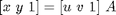Already we've encountered another common notational confusion. Sometimes a user will contact us and say "imtransform shrank my image in the horizontal direction, when it should have shrunk it in the vertical direction. Is this a bug in imtransform?" No, it's not a bug. The cause is that the user tried an A matrix from a source that defines the affine equation this way:
The A matrices in these two formulas are not the same; one is the transpose of the other.

Several common spatial transformations applied to images can be expressed in terms of an affine equation, including scaling:
Rotation:
And translation:
OK, enough background notation and equations. Next time we'll jump into MATLAB, construct some affine transformations, and apply them to points.

Published with MATLAB® 7.1

|

### コメント

コメントを残すには、ここ をクリックして MathWorks アカウントにサインインするか新しい MathWorks アカウントを作成します。